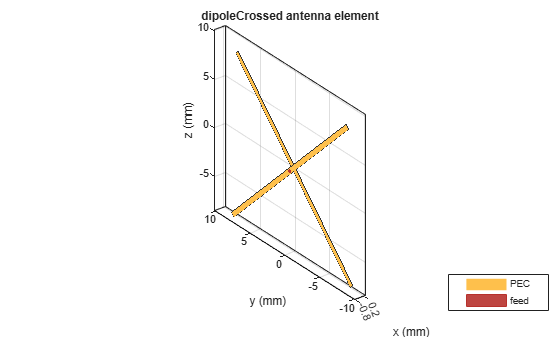# dipoleCrossed

Crossed dipole or turnstile antenna

Since R2019a

## Description

The `dipoleCrossed` object creates a turnstile antenna. By default, the turnstile antenna is center-fed and is on the Y-Z plane. This antenna operates at 6 GHz. You can also create a turnstile antenna using the following antenna elements: `bowtieTriangular`, `bowtieRounded`, and `dipoleBlade`.## Creation

### Syntax

``ant = dipoleCrossed``
``ant = dipoleCrossed(Name,Value)``

### Description

example

````ant = dipoleCrossed` creates a center-fed turnstile antenna operating at 6 GHz.```
````ant = dipoleCrossed(Name,Value)` sets properties using one or more name-value pairs. For example, `ant = dipoleCrossed('Element',dipoleBlade)` creates a turnstile antenna using a blade dipole antenna.```

## Properties

expand all

Antenna element to create a turnstile antenna, specified as an antenna object. You can also use the following antenna objects: `bowtieTriangular`, `bowtieRounded`, and `dipoleBlade`.

Example: `'Element',dipoleBlade`

Example: `ant.Element = dipoleBlade`

Data Types: `char` | `string`

Angles made by the antenna element arms with respect to the X-Y plane, specified as a two-element signed vector.

Example: `'ArmElevation',[50 -60]`

Example: `ant.ArmElevation = [50 -60]`

Data Types: `double`

Magnitude of voltage applied to the feeds, specified as a two-element vector with each element in volts.

Example: `'FeedVoltage',[2 2]`

Example: `ant.FeedVoltage = [2 2]`

Data Types: `double`

Phase shift applied to the voltage at the feeds, specified as a two-element vector with each element in degrees.

Example: `'FeedPhase',[0 50]`

Example: `ant.FeedPhase = [0 50]`

Data Types: `double`

Tilt angle of the antenna, specified as a scalar or vector with each element unit in degrees. For more information, see Rotate Antennas and Arrays.

Example: `Tilt=90`

Example: `Tilt=[90 90]`,`TiltAxis=[0 1 0;0 1 1]` tilts the antenna at 90 degrees about the two axes defined by the vectors.

Note

The `wireStack` antenna object only accepts the dot method to change its properties.

Data Types: `double`

Tilt axis of the antenna, specified as:

• Three-element vector of Cartesian coordinates in meters. In this case, each coordinate in the vector starts at the origin and lies along the specified points on the X-, Y-, and Z-axes.

• Two points in space, each specified as three-element vectors of Cartesian coordinates. In this case, the antenna rotates around the line joining the two points in space.

• A string input describing simple rotations around one of the principal axes, 'X', 'Y', or 'Z'.

Example: `TiltAxis=[0 1 0]`

Example: `TiltAxis=[0 0 0;0 1 0]`

Example: `TiltAxis = 'Z'`

Data Types: `double`

## Object Functions

 `show` Display antenna, array structures or shapes `axialRatio` Axial ratio of antenna `beamwidth` Beamwidth of antenna `charge` Charge distribution on antenna or array surface `current` Current distribution on antenna or array surface `design` Design prototype antenna or arrays for resonance around specified frequency `EHfields` Electric and magnetic fields of antennas; Embedded electric and magnetic fields of antenna element in arrays `impedance` Input impedance of antenna; scan impedance of array `mesh` Mesh properties of metal, dielectric antenna, or array structure `meshconfig` Change mesh mode of antenna structure `optimize` Optimize antenna or array using SADEA optimizer `pattern` Radiation pattern and phase of antenna or array; Embedded pattern of antenna element in array `patternAzimuth` Azimuth pattern of antenna or array `patternElevation` Elevation pattern of antenna or array `rcs` Calculate and plot radar cross section (RCS) of platform, antenna, or array `returnLoss` Return loss of antenna; scan return loss of array `sparameters` Calculate S-parameter for antenna and antenna array objects `vswr` Voltage standing wave ratio of antenna

## Examples

collapse all

Create and view a crossed dipole antenna with default property values.

`ant = dipoleCrossed`
```ant = dipoleCrossed with properties: Element: [1x1 dipole] ArmElevation: [45 -45] FeedVoltage: [1 1] FeedPhase: [0 90] Tilt: 0 TiltAxis: [1 0 0] ```
`show(ant)`## Version History

Introduced in R2019a